# Calculate the velocity when 2 progressive waves are added together

Bolter
Homework Statement:
Finding the resultant velocity of when 2 waves are added together
Relevant Equations:
See below
So here is my problem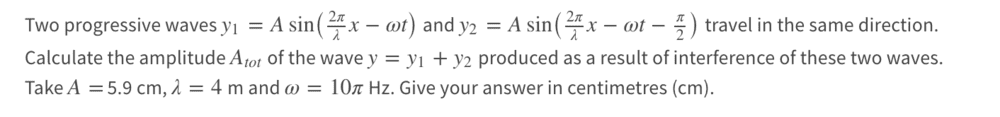I have had a go at this and get an answer of 8.34cm to be my final amplitude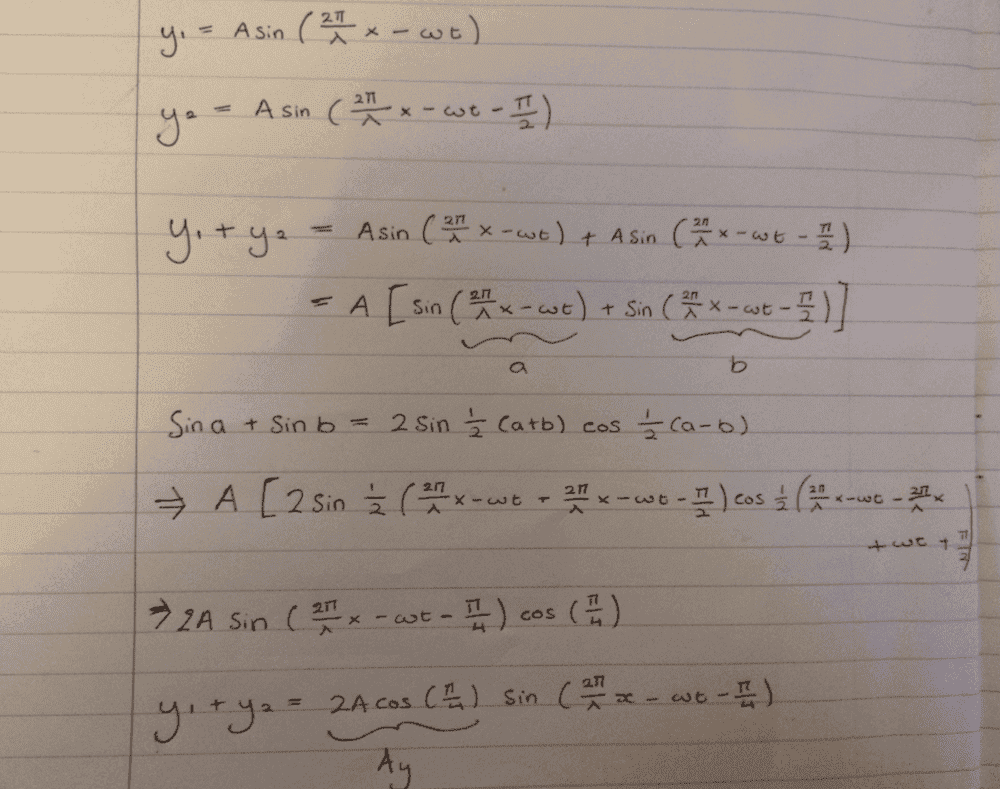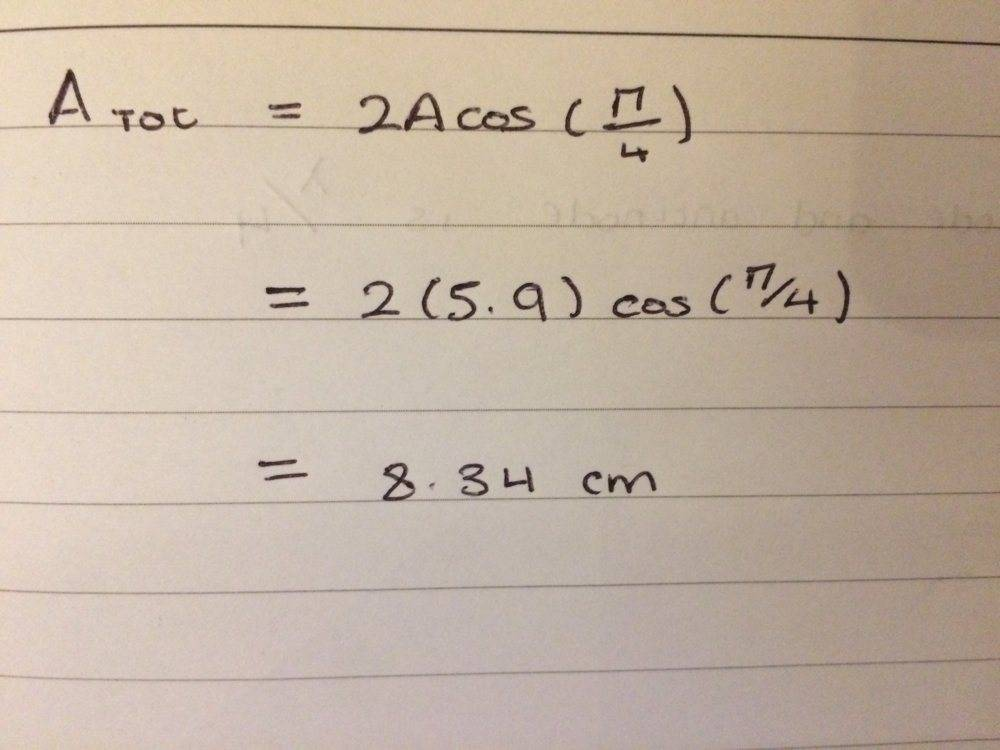The next part that I have been given is to calculate the velocity of when 2 waves are added together. I'm not so sure how to go about this, this is what I have tried anyway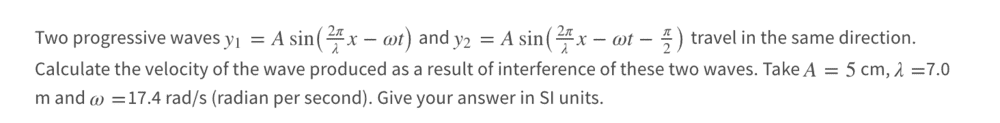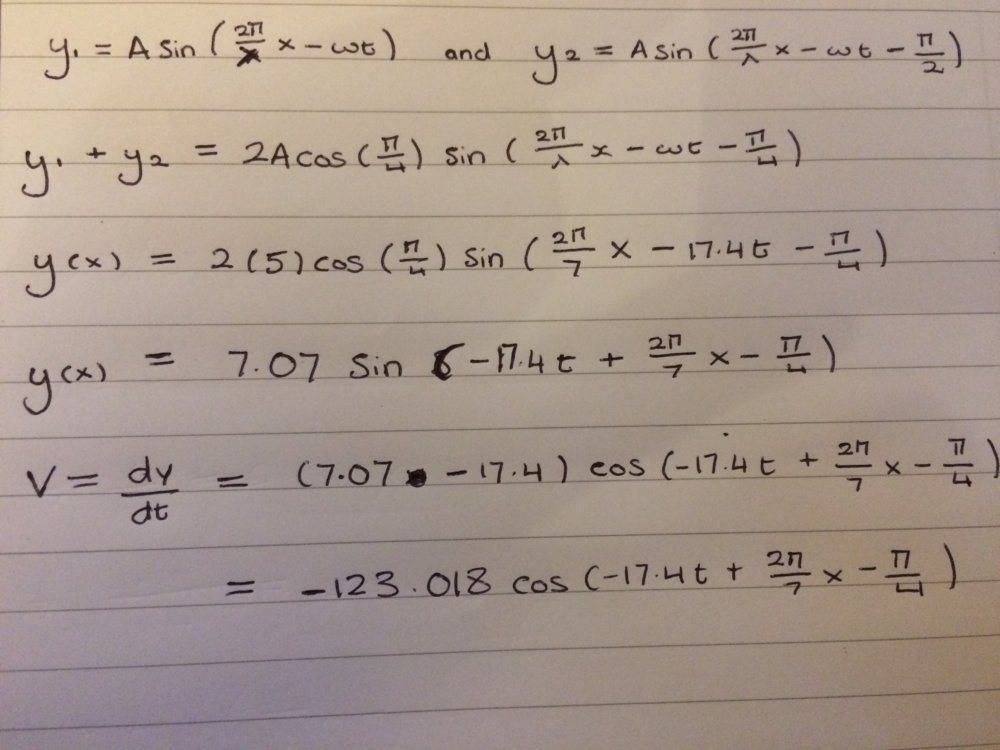Thanks!

Bolter
Could I just work out the velocity of the wave produced from doing this?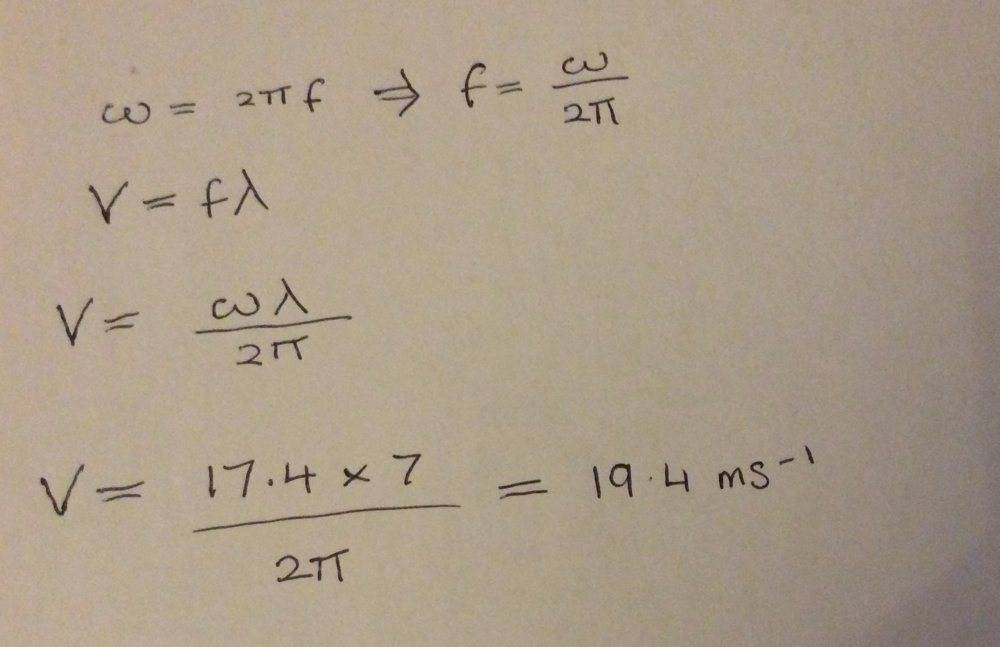I'm really confused here

Bolter
So I have thought about this question again, and I'm thinking that they are asking for the maximum velocity here of the wave that gets produced, since I cannot work out the velocity at any given time of the wave as I am not given a time value or the horizontal displacement x

So would this be an appropriate thing to do?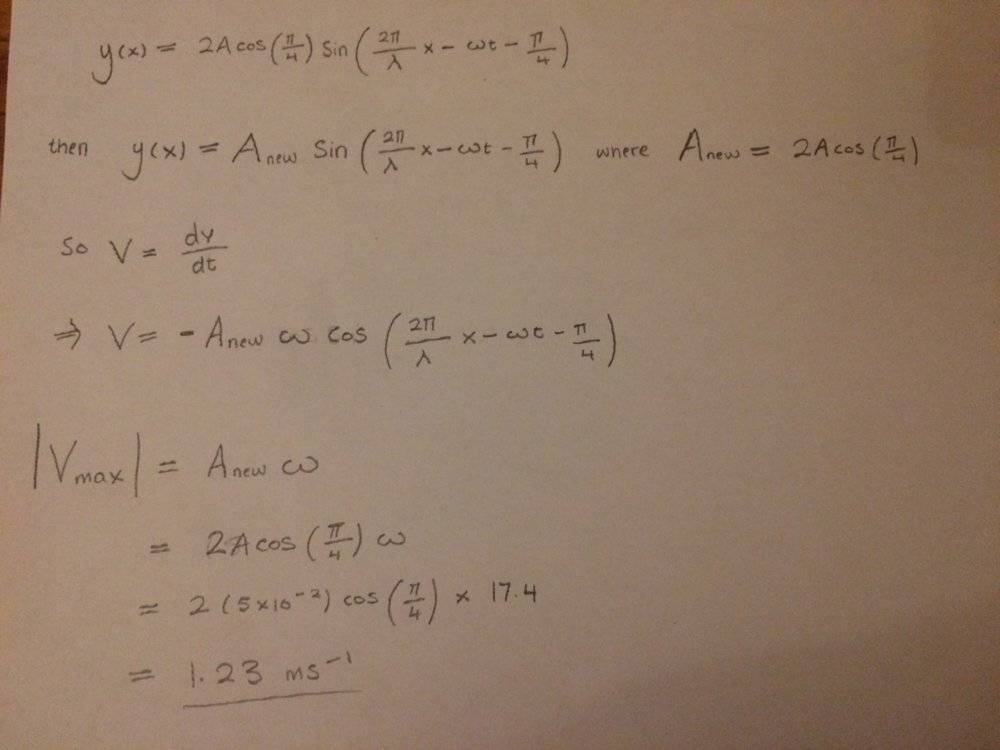Bolter
If a speed of 1.23 m/s is correct? Would it be best to include the negative sign as it asks for the velocity so I shouldn't be taking the absolute value of that then

Homework Helper
Gold Member
2022 Award
In post #3 you have calculated the max velocity at which a point on the wave rises. That is not the velocity of the wave.

Bolter
In post #3 you have calculated the max velocity at which a point on the wave rises. That is not the velocity of the wave.

Ok thank you, I was a bit overwhelmed by this question as they had given extra info that wasn't used in the calculation so I ought to think I was doing something wrong. I see my error here now. Thanks again!

•